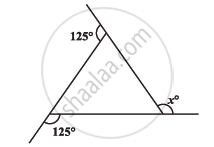# Find X In the Following Figures - Mathematics

Find x in the following figures#### Solution

We know that the sum of all exterior angles of any polygon is 360º.

125° + 125° + x = 360°

250° + x = 360°

x = 110°

Concept: Angle Sum Property of a Quadrilateral
Is there an error in this question or solution?
Chapter 3: Understanding Quadrilaterals - Exercise 3.2 [Page 44]

#### APPEARS IN

NCERT Class 8 Maths